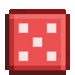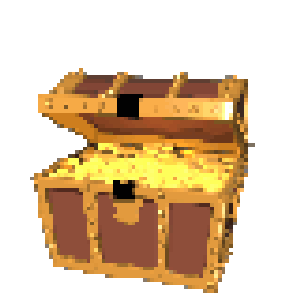## RANDOM NUMBERSGames are more interesting if they are different each time you play. In board games, rolled dice and shuffled cards are typical. In video games, random numbers are used. You can use the code random to "roll dice" and get a number between 1 and 6. The code below says to the computer, “Generate a number between 1 and 6. If you roll a 1, the player has found a dollar.”

``` ```

``````(set: \$diceroll to (random: 1, 6))
(if: \$diceroll is 1)[
You found a dollar! (set: \$money to \$money + 1)
]
``````
``` ```

The code below say to the computer, “Generate a number of coins (between 1 and 100) to be found in a treasure chest and tell the player how many coins they found.”

``` ```

``````(set: \$randomtreasure to (random: 1, 100))
You found \$randomtreasure coins in the treasure chest!
(set: \$coins to \$coins + \$randomtreasure)
``````
``` ```CONTINUE TO THE NEXT POST: Twine Music and Sound Effects from YouTube

RECENT POSTS